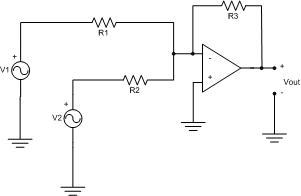Lab 2 Report ¨C DC and AC Analysis with PSpice Software

Bill Chun Wai Hung

29 June 2004

A. Describe the Set-up

There are two parts of the lab.

In Part I, the operational amplifier (op amp) is connected with only one voltage source. The connection is like the diagram in figure 1, expect there has only one input. The input resistance (R1) is 1k ohm, and the resistance across the op amp (R2) is 2k ohm. The voltage gain is

Gain = Vout/ Vin = - 2k/1k = -2Figure 1.

In Part II, the set-up is shown in figure 1. R1 and R2 is set to be 1k ohm, and R3 is 2k ohm. The output voltage (Vout) should be

Vout = -(R3/R2)V2 ¨C (R3/R1)V1

The diagram for the op amp circuit is:

B. Desbribe Inputs

C. Describe What you Observe

In part I, the input voltage is 4V (the signal generator is 2V peek-to-peek), so the expected output voltage (Vout) is 4V x 2 = 8V. The oscilloscope showed a peek-to-peek voltage of 8.109V, which is the experimental output voltage. The result is close to the expected output voltage (8V). The percentage difference is 1.36%.

Vin =4V, Gain = 2, Vout =8V

In part II, the first dc input voltage (V1) is 0.309V, and the second ac input voltage (V2) is 2V(the signal generator shows 1V), so because

Vout = -(R3/R2)V2 ¨C (R3/R1)V1

Where R1=1k, R2=1k, R3=2k

Therefore, the voltage out is expected to be around 2V x 2 = 4V. The oscilloscope showed a peek-to-peek voltage of 4.188V, which is the experimental output voltage. The result is close to the expected output voltage (4V). The percentage difference is 4.70%.

The first dc input voltage(V1) is set to be small, because V1 only gives an offset of the output voltage. When V1 is increased (say from 0.2V to 0.6V), the output voltage is wave form shifted upper. In similar case, when V1 is decreased (say from 0.6V to 0.2V), the output voltage wave form is shifted lower. This is because V1 is a dc input, and the dc input does not change the ac waveform, and the dc input only shift the output waveform upper or lower. In additional, when the waveform is shifted too high or too low, the waveform becomes a flat line. This is because the supply voltage of the op amp is not large enough to generate an output voltage that the input voltages (V1 and V2) generated. In other words, the supply voltage is not large enough to generate the required waveform.

D. What you can deduce

In this lab, an audio mixer is desired. The circuit should sum two inputs together. In the lab, it does show the input added together, because the dc voltage shifts the ac waveform generated in the oscilloscope (output).

What I am planning to do in order to claim my circuit works.

I am going to change my input voltage to other voltages. Like 2 V and 2V, and the supply voltage to higher voltages, like 12V. In this case, my circuit should add the voltages together. Because the gain is 2, so the output voltage should be 2V x 2 + 2V x 2 = 8V.